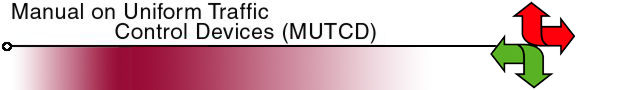Road Safety Performance Associated with Improved Traffic Signal Design and Increased Signal Conspicuity

7.0 Methodology to Estimate the Safety Improvement Effects

The method used in this study is based on the application of the claims prediction model in an Empirical Bayes (EB) approach, as described by Hauer (Hauer, 1997) and Sayed (Sayed, et al., 1998(2) and Sayed 1999). This approach is used to correct for the regression to the mean effects, an important consideration in road safety analysis. The methodology also uses claim and traffic volume data for a comparison group to represent the time trend from the before to after time periods. Therefore, it is important that the comparison group represents a random sample and not be selected because of high claim experience.

The reduction in the number of claims at the treatment sites (sites where the visibility of the signal backboard has been improved) can be calculated using the Odds Ratio (O.R.) according to equation (4). With the odd-ratio calculated, the effect of the treatment is determined by subtracting 1 from the odds ratio, as shown below.

(Equation 4)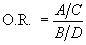TreatmentEffect = O.R. -1

where:
A = number of claims in the comparison group during the pre-improvement period.
B = the EB estimate of claims in the treatment site(s) had no treatment taken place.
C = number of claims in the comparison group during the post-improvement period.
D = number of claims in the treatment group during the post-improvement period.

It should be noted that all quantities in the Odds ratio are observed quantities (with assumed Poisson distribution), with the exception of quantity B, which is calculated. Therefore, the major work involved in evaluating the benefits of a certain treatment consists of determining the quantity B. This quantity is calculated by utilizing claim prediction models and the Empirical Bayes refinement procedure. Note that the theoretical background associated with the Empirical Bayes technique is beyond the scope of this paper and again, the reader is directed to road safety engineering research for greater detail of the Empirical Bayes approach.

The Empirical Bayes safety estimate and its variance are calculated using equations 5 and 6 as follows:

(Equation 5)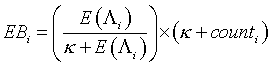(Equation 6)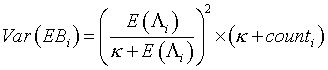where: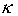= the negative binomial parameter of the claim prediction model.
counti = the observed claim frequency in the before period.

The value B in the Odds Ratio (equation 3) is calculated by using equation (6) (Sayed and de Leur, 2001).

(Equation 7)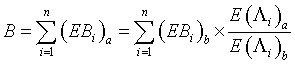where:
(EBi)a = the Empirical Bayes safety estimate of treated site i in the "after" period had no treatment taken place.
(EBi)b = the Empirical Bayes safety estimate of treated site i that occurred in the "before" period.
E(Λi)a = the claim frequency given by the claim prediction model for treated site i using its traffic flows in the "after" period.
E(Λi)b = the claim frequency given by the claim prediction model for treated site i using its traffic flows in the "before" period.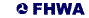United States Department of Transportation - Federal Highway Administration